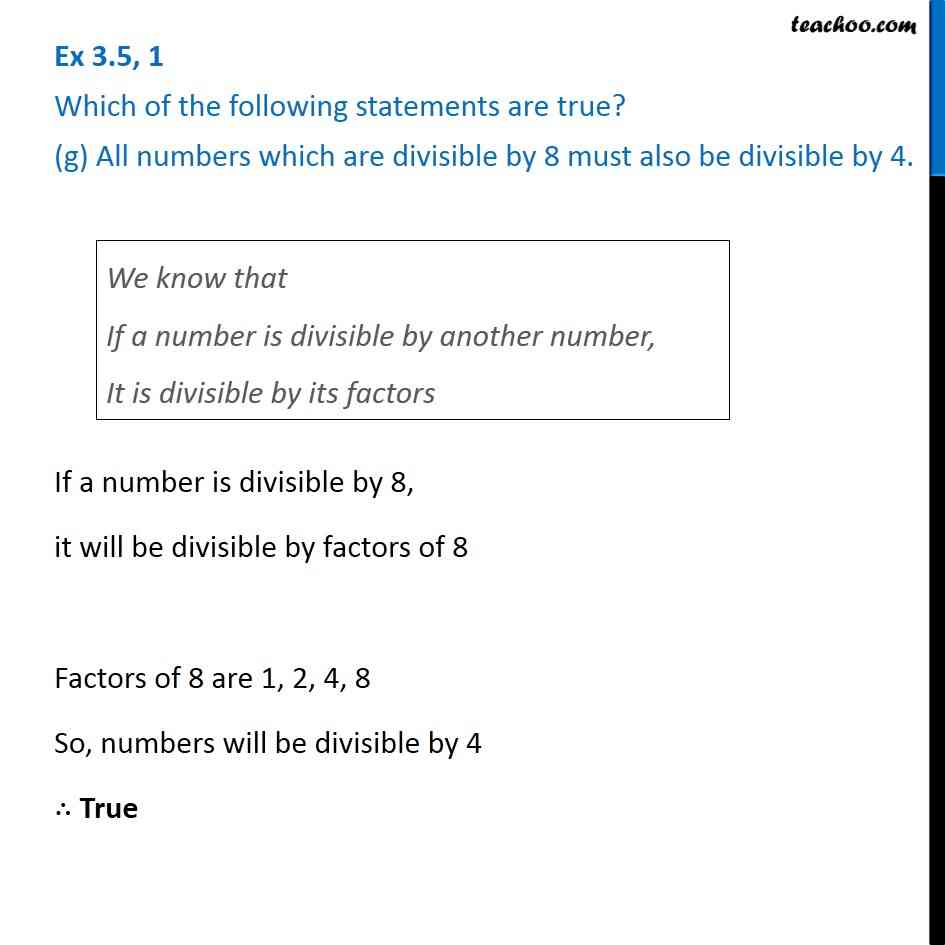Ex 3.5

Chapter 3 Class 6 Playing with Numbers
Serial order wiseLearn in your speed, with individual attention - Teachoo Maths 1-on-1 Class

### Transcript

Ex 3.5, 1 Which of the following statements are true? (g) All numbers which are divisible by 8 must also be divisible by 4.We know that If a number is divisible by another number, It is divisible by its factors If a number is divisible by 8, it will be divisible by factors of 8 Factors of 8 are 1, 2, 4, 8 So, numbers will be divisible by 4 ∴ True